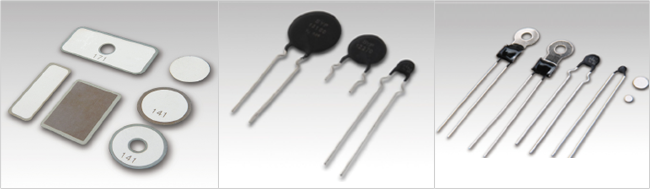## Definition

### 1. Resistance Temperature Characteristic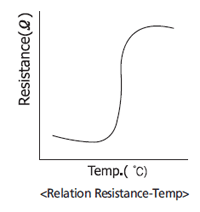Resistance temperature characteristic of PTC Thermistor shows that logarithm of resistance is proportional to temperature. Some abruptly increase the resistance at the gradient of 15 to 60%/℃ when reaching the abruptresistance change temperature, while others indicate relatively gradual resistance temperature gradient. Resistance rises abruptly from a certain temperature. The abrupt resistance change temperature ranges from 30 to 320℃.

### 2. Voltage-Current Characteristic (static characteristic)1. PTC Thermistor shows a self-heating function when voltage     is applied. When operating point exceeds the maximum     current point, the current becomes constant and PTC Thermistor itself     automatically regulates temperature. This provides PTC Thermistor     PTC Thermistor with a wide range of applications as a constant     temperature heating element. 2. When operating point is below the maximum current point,     PTC Thermistor shows over current limiting function.
When something unusual occurs in the electronic circuit and over current flows to PTC Thermistor, PTC Thermistor can limit the current in the circuit by shifting the operating point beyond the current maximum point. Coefficient of PTC Thermistor's thermal dissipation varies with the change of ambient conditions. Thus, the operating point of PTC Thermistor is shifted and resistance value varies. This characteristic can be used for the level gage, etc. by sensing the shift of the operating point.
Voltage-current characteristic (static characteristic) varies with the values of parallel and series resistance.
The proper selection of series and parallel resistance values will provide a circuit having constant current characteristic
within a given voltage range. Load voltage or load current can be kept constant irrespective of the fluctuation of power voltage or load resistance by inserting series or parallel in circuit. Furthermore, the proper combination of more than two PTC Thermistors having different static characteristic will extend the range of load voltage or load current kept in constant.

### 3. Current-time Characteristic (Dynamic Characteristic)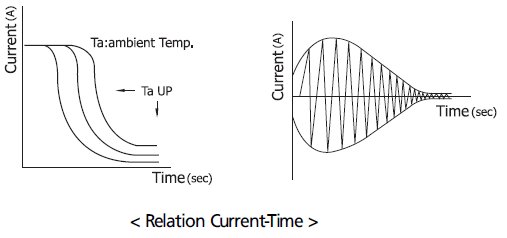When more than a given voltage is applied to PTC Thermistor, large current flows in the instant of voltage application,
due to low resistance. However, with the lapse of time, resistance value increases and the current decreases by PTC
Thermistor's self-heating function. The insertion of fixed resistance in series or in parallel will change PTC Thermistor's
characteristic.

## Products & Applications

1

PTC Thermistor

Heating Element•Self-Regulating Heating
•High-Reliability
•Stability
•Permanently Available

#### 2) Applications2

PTC Thermister

Over Current Protection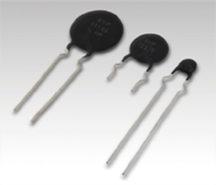•Inrush Current Limiter
•Circuit Protection
•Inrush Current Limiter
•Circuit Protection

#### 2) Applications3

PTC Theremister

Over Temperature Protection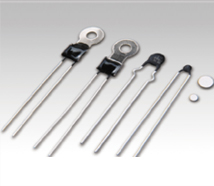•Small Size
•Quick Response Time
•Accurate and Automatic
•Temperature Management